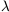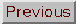### COSMOLOGICAL CONSTANT ()

Adapted from P. Coles, 1999, The Routledge Critical Dictionary of the New Cosmology, Routledge Inc., New York. Reprinted with the author's permission. To order this book click here: http://www.routledge-ny.com/books.cfm?isbn=0415923549

A constant originally introduced into the equations of general relativity by Albert Einstein himself in order to produce a cosmological model which discovery by Edwin Hubble of the expansion of the Universe. Later events caused Einstein to regret the addition of this term, but its legacy still lingers.

What Einstein did was to modify the left hand side of the field equations (see Einstein equations) by changing the terms that involve the curvature of spacetime. This was tantamount to modifying the law of gravity slightly so that on sufficiently large scales he could balance the universal attraction of gravity with a repulsive force of his own devising. The effect of the cosmological constanton Newton's law for the gravitational force between two masses is to add a new term to the usual law (which depends on the inverse square of the separation of masses); the new term is directly proportional to the separation, instead of depending on the inverse square. On large scales, therefore, the-term dominates. If it is positive,can be understood as a cosmic repulsion; if it is negative, it acts as a cosmic tension leading to an extra attraction over and above the usual gravitational force. This modification was not entirely arbitrary, however, because it is completely consistent with the fundamental reasoning that led Einstein to general relativity in the first place.

One might imagine that the cosmological constant would have vanished from the scene with the discovery of the expansion of the Universe, but that certainly did not happen. With developments in the Big Bang theory, cosmologists began to ponder the consequences of fundamental interactions in the very early Universe. These considerations concerned the right-hand-side of Einstein's equations, the part of the theory of general relativity that deals with the properties of matter. It was realised that the cosmological constant term could just as easily be put on the left-hand side of the field equations. The cosmological constant is a vacuum energy density: an energy not directly associated with matter or radiation, but with `empty' space.

Each time matter changes state (i.e. each time it undergoes a phase transition), some amount of vacuum energy is expected to remain. Physicists working on the theory of elementary particles tried to calculate the net amount of vacuum energy produced by all the phase transitions the Universe underwent as it cooled. The answer is catastrophically large: about 10120 times larger than the density of all the matter in the Universe. Such a result is at odds with observations, to put it mildly.

Some cosmologists believe that a cosmological constant term is necessary in order to reconcile the age of the Universe with estimates of the Hubble constant and the density parameter. But the size of the term required corresponds to a vacuum energy density of the same order as the density of matter, about 10120 times smaller than the predictions of particle physics. Many are deeply uncomfortable about the size of this discrepancy, and suggest that it means that the cosmological constant has to be exactly zero.

The cosmological constant also plays a role in the inflationary Universe. The mathematical solution that describes a universe undergoing inflation, first found by Willem de Sitter in 1917, involves the cosmological constant term (actually that is all it involves - the de Sitter universe is empty apart from the vacuum density). The scalar field responsible for driving the inflationary expansion behaves in such a way that the vacuum energy dominates: the solution in this case is identical to the de Sitter model.

FURTHER READING:

Einstein, A., `Cosmological considerations on the general theory of relativity', 1917, reprinted in The Principle of Relativity, edited by H.A. Lorentz et al., (Dover, New York, 1950). Weinberg, S. `The cosmological constant problem', Reviews of Modern Physics, 1989, 68, 1. Goldsmith, D., Einstein's Greatest Blunder? The Cosmological Constant and Other Fudge Factors in the Physics of the Universe (Harvard University Press, Cambridge, MA, 1995).